# Using logical functions in Excel: AND, OR, XOR and NOT

The tutorial explains the essence of Excel logical functions AND, OR,  XOR and NOT and provides formula examples that demonstrate their common and inventive uses.

Last week we tapped into the insight of Excel logical operators that are used to compare data in different cells. Today, you will see how to extend the use of logical operators and construct more elaborate tests to perform more complex calculations. Excel logical functions such as AND, OR,  XOR and NOT will help you in doing this.

## Excel logical functions - overview

Microsoft Excel provides 4 logical functions to work with the logical values. The functions are AND, OR,  XOR and NOT. You use these functions when you want to carry out more than one comparison in your formula or test multiple conditions instead of just one. As well as logical operators, Excel logical functions return either TRUE or FALSE when their arguments are evaluated.

The following table provides a short summary of what each logical function does to help you choose the right formula for a specific task.

 Function Description Formula Example Formula Description AND Returns TRUE if all of the arguments evaluate to TRUE. `=AND(A2>=10, B2<5)` The formula returns TRUE if a value in cell A2 is greater than or equal to 10, and a value in B2 is less than 5, FALSE otherwise. OR Returns TRUE if any argument evaluates to TRUE. `=OR(A2>=10, B2<5)` The formula returns TRUE if A2 is greater than or equal to 10 or B2 is less than 5, or both conditions are met. If neither of the conditions it met, the formula returns FALSE. XOR Returns a logical Exclusive Or of all arguments. `=XOR(A2>=10, B2<5)` The formula returns TRUE if either A2 is greater than or equal to 10 or B2 is less than 5. If neither of the conditions is met or both conditions are met, the formula returns FALSE. NOT Returns the reversed logical value of its argument. I.e. If the argument is FALSE, then TRUE is returned and vice versa. `=NOT(A2>=10)` The formula returns FALSE if a value in cell A1 is greater than or equal to 10; TRUE otherwise.

In additions to the four logical functions outlined above, Microsoft Excel provides 3 "conditional" functions - IF, IFERROR and IFNA.

#### Excel logical functions - facts and figures

1. In arguments of the logical functions, you can use cell references, numeric and text values, Boolean values, comparison operators, and other Excel functions. However, all arguments must evaluate to the Boolean values of TRUE or FALSE, or references or arrays containing logical values.
2. If an argument of a logical function contains any empty cells, such values are ignored. If all of the arguments are empty cells, the formula returns #VALUE! error.
3. If an argument of a logical function contains numbers, then zero evaluates to FALSE, and all other numbers including negative numbers evaluate to TRUE. For example, if cells A1:A5 contain numbers, the formula =AND(A1:A5) will return TRUE if none of the cells contains 0, FALSE otherwise.
4. A logical function returns the #VALUE! error if none of the arguments evaluate to logical values.
5. A logical function returns the #NAME? error if you've misspell the function's name or attempted to use the function in an earlier Excel version that does not support it. For example, the XOR function can be used in Excel 2016 and 2013 only.
6. In Excel 2016, 2013, 2010 and 2007, you can include up to 255 arguments in a logical function, provided that the total length of the formula does not exceed 8,192 characters. In Excel 2003 and lower, you can supply up to 30 arguments and the total length of your formula shall not exceed 1,024 characters.

## Using the AND function in Excel

The AND function is the most popular member of the logic functions family. It comes in handy when you have to test several conditions and make sure that all of them are met. Technically, the AND function tests the conditions you specify and returns TRUE if all of the conditions evaluate to TRUE, FALSE otherwise.

The syntax for the Excel AND function is as follows:

AND(logical1, [logical2], …)

Where logical is the condition you want to test that can evaluate to either TRUE or FALSE. The first condition (logical1) is required, subsequent conditions are optional.

And now, let's look at some formula examples that demonstrate how to use the AND functions in Excel formulas.

 Formula Description `=AND(A2="Bananas", B2>C2)` Returns TRUE if A2 contains "Bananas" and B2 is greater than C2, FALSE otherwise. `=AND(B2>20, B2=C2)` Returns TRUE if B2 is greater than 20 and B2 is equal to C2, FALSE otherwise. `=AND(A2="Bananas", B2>=30, B2>C2)` Returns TRUE if A2 contains "Bananas", B2 is greater than or equal to 30 and B2 is greater than C2, FALSE otherwise.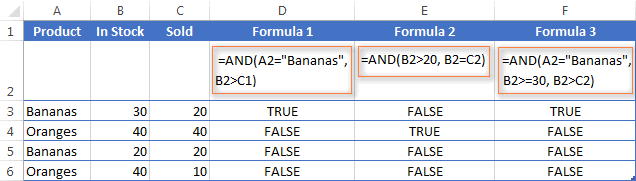#### Excel AND function - common uses

By itself, the Excel AND function is not very exciting and has narrow usefulness. But in combination with other Excel functions, AND can significantly extend the capabilities of your worksheets.

One of the most common uses of the Excel AND function is found in the logical_test argument of the IF function to test several conditions instead of just one. For example, you can nest any of the AND functions above inside the IF function and get a result similar to this:

`=IF(AND(A2="Bananas", B2>C2), "Good", "Bad")`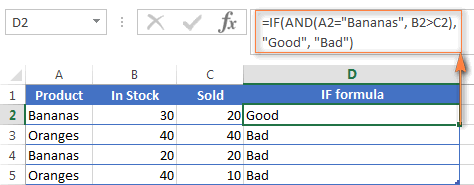For more IF / AND formula examples, please check out his tutorial: Excel IF function with multiple AND conditions.

#### An Excel formula for the BETWEEN condition

If you need to create a between formula in Excel that picks all values between the given two values, a common approach is to use the IF function with AND in the logical test.

For example, you have 3 values in columns A, B and C and you want to know if a value in column A falls between B and C values. To make such a formula, all it takes is the IF function with nested AND and a couple of comparison operators:

Formula to check if X is between Y and Z, inclusive:

`=IF(AND(A2>=B2,A2<=C2),"Yes", "No")`

Formula to check if X is between Y and Z, not inclusive:

`=IF(AND(A2>B2, A2<C2),"Yes", "No")`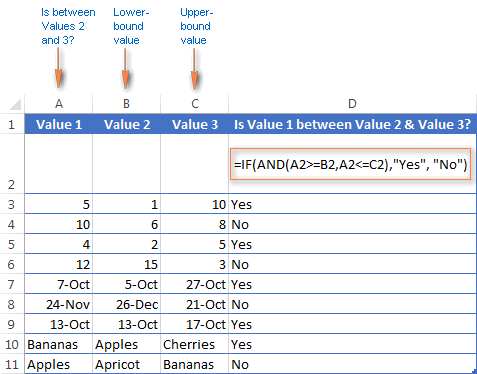As demonstrated in the screenshot above, the formula works perfectly for all data types - numbers, dates and text values. When comparing text values, the formula checks them character-by-character in the alphabetic order. For example, it states that Apples in not between Apricot and Bananas because the second "p" in Apples comes before "r" in Apricot. Please see Using Excel comparison operators with text values for more details.

As you see, the IF /AND formula is simple, fast and almost universal. I say "almost" because it does not cover one scenario. The above formula implies that a value in column B is smaller than in column C, i.e. column B always contains the lower bound value and C - the upper bound value. This is the reason why the formula returns "No" for row 6, where A6 has 12, B6 - 15 and C6 - 3 as well as for row 8 where A8 is 24-Nov, B8 is 26-Dec and C8 is 21-Oct.

But what if you want your between formula to work correctly regardless of where the lower-bound and upper-bound values reside? In this case, use the Excel MEDIAN function that returns the median of the given numbers (i.e. the number in the middle of a set of numbers).

So, if you replace AND in the logical test of the IF function with MEDIAN, the formula will go like:

`=IF(A2=MEDIAN(A2:C2),"Yes","No")`

And you will get the following results: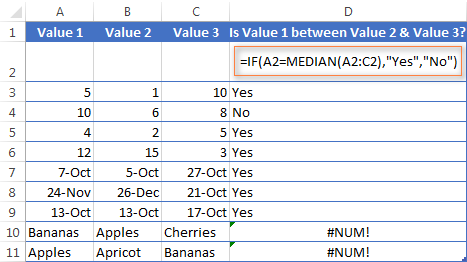As you see, the MEDIAN function works perfectly for numbers and dates, but returns the #NUM! error for text values. Alas, no one is perfect : )

If you want a perfect Between formula that works for text values as well as for numbers and dates, then you will have to construct a more complex logical text using the AND / OR functions, like this:

`=IF(OR(AND(A2>B2, A2<C2), AND(A2<B2, A2>C2)), "Yes", "No")`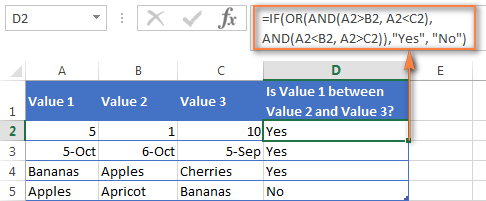## Using the OR function in Excel

As well as AND, the Excel OR function is a basic logical function that is used to compare two values or statements. The difference is that the OR function returns TRUE if at least one if the arguments evaluates to TRUE, and returns FALSE if all arguments are FALSE. The OR function is available in all versions of Excel 2016 - 2000.

The syntax of the Excel OR function is very similar to AND:

OR(logical1, [logical2], …)

Where logical is something you want to test that can be either TRUE or FALSE. The first logical is required, additional conditions (up to 255 in modern Excel versions) are optional.

And now, let's write down a few formulas for you to get a feel how the OR function in Excel works.

 Formula Description `=OR(A2="Bananas", A2="Oranges")` Returns TRUE if A2 contains "Bananas" or "Oranges", FALSE otherwise. `=OR(B2>=40, C2>=20)` Returns TRUE if B2 is greater than or equal to 40 or C2 is greater than or equal to 20, FALSE otherwise. `=OR(B2=" ", C2="")` Returns TRUE if either B2 or C2 is blank or both, FALSE otherwise.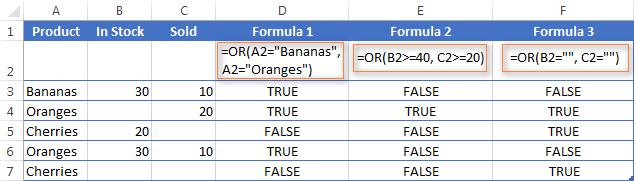As well as Excel AND function, OR is widely used to expand the usefulness of other Excel functions that perform logical tests, e.g. the IF function. Here are just a couple of examples:

IF function with nested OR

`=IF(OR(B2>30, C2>20), "Good", "Bad")`

The formula returns "Good" if a number in cell B3 is greater than 30 or the number in C2 is greater than 20, "Bad" otherwise.

Excel AND / OR functions in one formula

Naturally, nothing prevents you from using both functions, AND & OR, in a single formula if your business logic requires this. There can be infinite variations of such formulas that boil down to the following basic patterns:

`=AND(OR(Cond1, Cond2), Cond3)`

`=AND(OR(Cond1, Cond2), OR(Cond3, Cond4)`

`=OR(AND(Cond1, Cond2), Cond3)`

`=OR(AND(Cond1,Cond2), AND(Cond3,Cond4))`

For example, if you wanted to know what consignments of bananas and oranges are sold out, i.e. "In stock" number (column B) is equal to the "Sold" number (column C), the following OR/AND formula could quickly show this to you:

`=OR(AND(A2="bananas", B2=C2), AND(A2="oranges", B2=C2))`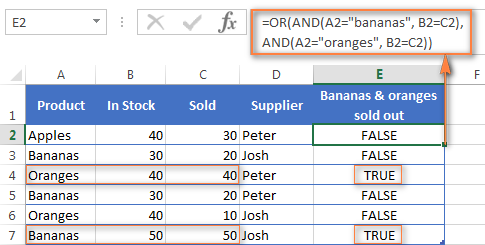OR function in Excel conditional formatting

`=OR(\$B2="", \$C2="")`

The rule with the above OR formula highlights rows that contain an empty cell either in column B or C, or in both.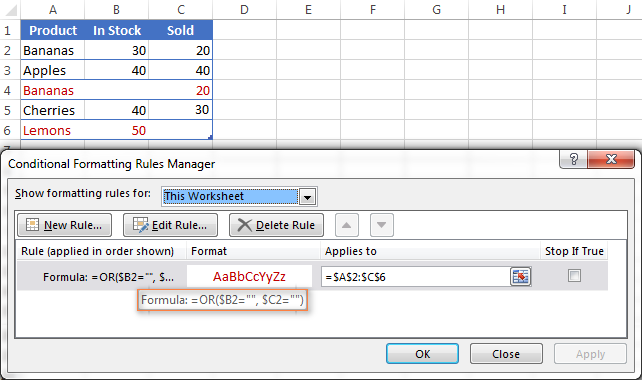## Using the XOR function in Excel

In Excel 2013, Microsoft introduced the XOR function, which is a logical Exclusive OR function. This term is definitely familiar to those of you who have some knowledge of any programming language or computer science in general.  For those who don't, the concept of 'Exclusive Or' may be a bit difficult to grasp at first, but hopefully the below explanation illustrated with formula examples will help.

The syntax of the XOR function is identical to OR's :

XOR(logical1, [logical2],…)

The first logical statement (Logical 1) is required, additional logical values are optional. You can test up to 254 conditions in one formula, and these can be logical values, arrays, or references that evaluate to either TRUE or FALSE.

In the simplest version, an XOR formula contains just 2 logical statements and returns:

• TRUE if either argument evaluates to TRUE.
• FALSE if both arguments are TRUE or neither is TRUE.

This might be easier to understand from the formula examples:

 Formula Result Description `=XOR(1>0, 2<1)` TRUE Returns TRUE because the 1st argument is TRUE and the 2nd argument is FALSE. `=XOR(1<0, 2<1)` FALSE Returns FALSE because both arguments are FALSE. `=XOR(1>0, 2>1)` FALSE Returns FALSE because both arguments are TRUE.

When more logical statements are added, the XOR function in Excel results in:

• TRUE if an odd number of the arguments evaluate to TRUE;
• FALSE if is the total number of TRUE statements is even, or if all statements are FALSE.

The screenshot below illustrates the point: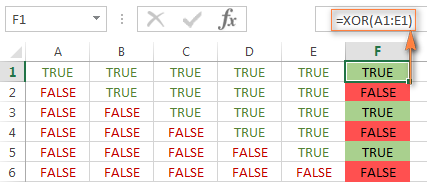If you are not sure how the Excel XOR function can be applied to a real-life scenario, consider the following example. Suppose you have a table of contestants and their results for the first 2 games. You want to know which of the payers shall play the 3rd game based on the following conditions:

• Contestants who won Game 1 and Game 2 advance to the next round automatically and don't have to play Game 3.
• Contestants who lost both first games are knocked out and don't play Game 3 either.
• Contestants who won either Game 1 or Game 2 shall play Game 3 to determine who goes into the next round and who doesn't.

A simple XOR formula works exactly as we want:

`=XOR(B2="Won", C2="Won")`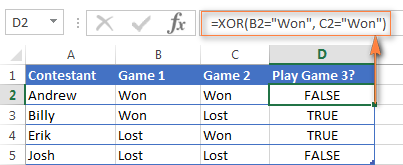And if you nest this XOR function into the logical test of the IF formula, you will get even more sensible results:

`=IF(XOR(B2="Won", C2="Won"), "Yes", "No")`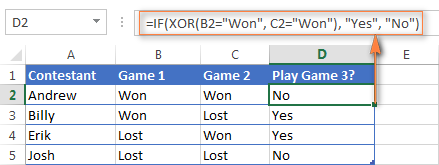## Using the NOT function in Excel

The NOT function is one of the simplest Excel functions in terms of syntax:

NOT(logical)

You use the NOT function in Excel to reverse a value of its argument. In other words, if logical evaluates to FALSE, the NOT function returns TRUE and vice versa. For example, both of the below formulas return FALSE:

`=NOT(TRUE)`

`=NOT(2*2=4)`

Why would one want to get such ridiculous results? In some cases, you might be more interested to know when a certain condition isn't met than when it is. For example, when reviewing a list of attire, you may want to exclude some color that does not suit you.  I'm not particularly fond of black, so I go ahead with this formula:

`=NOT(C2="black")`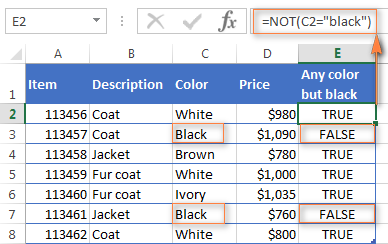As usual, in Microsoft Excel there is more than one way to do something, and you can achieve the same result by using the Not equal to operator: =C2<>"black".

If you want to test several conditions in a single formula, you can use NOT in conjunctions with the AND or OR function. For example, if you wanted to exclude black and white colors, the formula would go like:

`=NOT(OR(C2="black", C2="white"))`

And if you'd rather not have a black coat, while a black jacket or a back fur coat may be considered, you should use NOT in combination with the Excel AND function:

`=NOT(AND(C2="black", B2="coat"))`

Another common use of the NOT function in Excel is to reverse the behavior of some other function.  For instance, you can combine NOT and ISBLANK functions to create the ISNOTBLANK formula that Microsoft Excel lacks.

As you know, the formula =ISBLANK(A2) returns TRUE of if the cell A2 is blank. The NOT function can reverse this result to FALSE: =NOT(ISBLANK(A2))

And then, you can take a step further and create a nested IF statement with the NOT / ISBLANK functions for a real-life task:

`=IF(NOT(ISBLANK(C2)), C2*0.15, "No bonus :(")`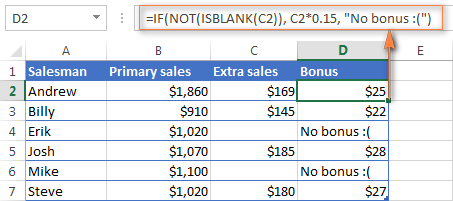Translated into plain English, the formula tells Excel to do the following. If the cell C2 is not empty, multiply the number in C2 by 0.15, which gives the 15% bonus to each salesman who has made any extra sales. If C2 is blank, the text "No bonus :(" appears.

In essence, this is how you use the logical functions in Excel. Of course, these examples have only scratched the surface of AND, OR, XOR and NOT capabilities. Knowing the basics, you can now extend your knowledge by tackling your real tasks and writing smart elaborate formulas for your worksheets.

## You may also be interested in

### 459 responses to "Using logical functions in Excel: AND, OR, XOR and NOT"

1.203 says:

Hi all,

I need assistance with the following conditions for a sales model.

The user wants to sell widgets and they do not want be charged for the 1st 5000 sales. They will then be billed \$20 for each widget over 5000 sales. They also only want to pay for a maximum number of widgets (10,000) even if sales exceed this level.
Any assistance would be greatly appreciated.

2.Bruno says:

This article was extremely well-made and useful. Thanks! One question tho, when using a formula with the same format as =OR(\$C1"", \$D2"") , could I instead do =OR(C1:C2"") ?

3.Jennifer says:

If cell B2 = 43830 (which is December 31, 2019 and assuming that cell B2 does say December 31, 2019) and if (-5 + 2 +7)>0 which is also true, how do I write this to make it work in an IF/AND statement?

Any help is Greatly appreciated!

4.wenz says:

I need help please, i need to a formula about this problem, from jan. 26,2019 to feb. 25, 2019 there are four fridays and 27 working days. The thing i need is on calculating working days minus the working hours for the whole month, see below example
BG5 BH5 BI5
Total Working Hrs Total Regular Hrs Total Overtime Hrs
is from jan26 to feb25 is only 260 hrs is what i also need formula
and the january month has 31 therefore the total days until february 25 is also 31 days but i need only 26 working days and 4 days fridays. I tried the formula for total overtime as this:=IF(BG5=>BH5,BG5-BH5,0) it only applies when there is only 30 days a month and 4 fridays but does not gave me when there are 5 fridays in a month and also if there is 31th day of the month.
Any help is Greatly appreciated!

5.cardo says:

Does RIGHT,LEN,FALSE belong to the logical category

•Svetlana Cheusheva (Ablebits.com Team) says:

Hi!

FALSE is categorized under Logical functions; RIGHT and LEN belong to the category of Text functions.

6.Cholly says:

thanks this note are very helpful to me as a student

7.neha says:

=IF(AND(E3<6,OR(TIME(11,45,0)<F3<TIME(12,0,0),TIME(18,45,0)<F3<TIME(19,30,0))),"early bird", "")

write a logical function to find out whether a customer is an early bird. (refer to the 'Early Bird' criteria stated above) In your function, you will have to reference Column E values (day of week) and Column F (time of order) to determine whether customers are early birds. Think about how you will reference the early bird criteria.
E3= weekday number (1-7)
f3 time 7:12pm
Early Bird criteria - Customers who want to avail of an Early Bird discount must place their orders on weekdays between 11:45 a.m. - 12:00 p.m. (for lunch) or 6:45 p.m. - 7:30 p.m. (for dinner).

can somebody help,

•Alexander Trifuntov (Ablebits.com Team) says:

Hello Neha!
If I understand your task correctly, the following formulas should work for you:

=IF(AND(E3 < 6,OR(AND(TIME(11,45,0) < F3, F3 < TIME(12,0,0)), AND(TIME(18,45,0) < F3,F3 < TIME(19,30,0)))), "early bird", "")

Hope you’ll find this information helpful.

8.Jane Soria says:

CURR. T. INV. VALUE VALUE CONVERTED IN AED
USD 11,545.00 (CURRENCY(1)=VALUE IN AED) * INV VALUE = TOTAL CONVERSION VALUE
EUR 1,199.00
KWD 2,000.00
AED 5,000.00
QAT 12,000.00
GBP 1,500.00

USD 3.674
EUR 3.99
KWD 11.9
AED 1
QAT 1.01
GBP 4.59

Can anyone help me with the formula for the above.
If I enter the currency whether it's Eur or USD the amount will be converted automatically and whatever the conversion is, it will be multiplied by Column 2 (Total Value) and the total conversion will be on column 3.

Appreciate you help.

•Alexander Trifuntov (Ablebits.com Team) says:

Hello Jane!
To show the rate for a necessary currency in cell C2, you can use this formula:

=VLOOKUP(A2,\$A\$10:\$B\$15,2,0)

\$A\$10:\$B\$15 is your table with the currency rate, A2 is a cell where the currency is specified (USD in your case).
If you have any additional questions, I will be happy to answer them.

9.Sanjoy Dutta says:

I want in excel sheet, 5+6+7*+8= 26. (*) it's cricket talk Batsman Not Out.
How I put in excel sheet.

•Alexander Trifuntov (Ablebits.com Team) says:

Hello Sanjoy!
I’m sorry but your task is not entirely clear to me. Could you please describe it in more detail? What is "cricket talk Batsman Not Out"?? Also write what exactly you want to receive.I will try to help you.

10.Miles says:

Help. I have a working formula like this, OR(D9="X", E9="55",D9="B"), in the data validation. How can I modify this in a way that when D9="B" then only "55" value is allowed in E9? meanwhile allowing any values on E9 when D9="X"?

•Alexander Trifuntov (Ablebits.com Team) says:

Hello Miles!
If I understand your task correctly, the following formula should work for you:

OR(AND(E9="55",D9="B"),D9="X")

11.Luminita says:

1. Having a personal ID number starting 1 or 5 for boys and 2 or 6 for girls, I must write in a column if that person is a girl or a boy. I tried to combine IF and XOR functions but didn`t work for me.
2. how to transform the numbers like 6,05 into text in this format: (six,05%)

Congratulations for what you are doing in helping us!

•Alexander Trifuntov (Ablebits.com Team) says:

Hello!

=CHOOSE(--LEFT(A1,1),"Boy","Girl", "","","Boy","Girl","","","")

and

=CHOOSE(--LEFT(A6,1),"one","two","three", "four","five","six","seven", "eight","nine")& MID(A6,SEARCH(",",A6,1),10)&"%"

I hope this will help

12.Nawab Hujjahtullah says:

Need result as below
1. If value is > or = 0 then result will be= 80,
2. If value is <0 to -03 then result will be= 70,
3. if value is -04 to -07 then result will be= 60
4. if value is -08 to -15 then result will be= 50

•Alexander Trifuntov (Ablebits.com Team) says:

Hello!
There is no need to use the AND or OR function.
The formula below will do the trick for you:

=IF(A1>=0,80,IF(A1>-3,70, IF(A1>-7,60,IF(A1>-15,50,"" ) )) )

•Dr Leigh Cassidy says:

Hi I have a similar problem to the one above but I need to make the values specific to individual suppliers
e.g IF "ACE" is the supplier but I need to specify tariffs for different pipe sizes eg <20, 20-25,40-45,50-63 etc but different suppliers have different names, size ranges and tariffs how can I write this so the correct tariff is selected for each pipe size in each supplier?
I would greatly appreciate some help with this!

•Alexander Trifuntov (Ablebits.com Team) says:

Hello!
You need to search by two criteria - the name of the supplier and the size of the pipe. Perhaps this article on searching by several criteria using the INDEX + MATCH functions will be useful
I hope this will help, otherwise please do not hesitate to contact me anytime.

•Dr Leigh Cassidy says:

Fantastic, thank you very much. I will look at the article just now. I may be back for more help....
Kind regards
Leigh

13.Phil Goodall says:

I have a more complicated logic function than I can work out. I am assessing building capacity and need to identify a building as 'under capacity' (where room capacity is greater than customer numbers), 'extend hours' (where room capacity is less than customer numbers, but where opening hours are equal to or less than 37.5 hours per week) and 'exceeds capacity' (where room capacity is less than customer numbers, but where opening hours are more than 37.5 hours per week OR where the room capacity is identified as 'zero').

•Alexander Trifuntov (Ablebits.com Team) says:

Hello Phil!
If I understand your task correctly, the following formula should work for you:

=IF(F1 > F2,"under capacity",IF(F3 < 37.5,"extend hours","zero"))

where
F1 - room capacity
F2 - customer numbers
F3 - opening hours

14.Paul says:

how to calculate for 3 of more arguments?
below is what I mean:
Grater or equator 100 =excellent
Between 50 to 90 =very good
Less than 40 =fail

•Alexander Trifuntov (Ablebits.com Team) says:

Hello!
The formula below will do the trick for you:

=IF(A1>=100,"excellent",IF(AND(A1>=50,A1<=90),"very good",IF(A1<40,"fail","")))

I hope this will help

15.Edoardo says:

Let's see if I can explain my problem:
I have a row of an approx 200 empty cells and I want to assign a number (1) at a defined interval (10.26). The formula I'm working on is the following

IF (10.26-(C1+R1)0.49; 1, 0)

My question is: there is a way to nest the two functions and make the SUM work dinamically maybe using a different formula altogether?

•Alexander Trifuntov (Ablebits.com Team) says:

Hello!
I’m sorry but your task is not entirely clear to me. Could you please describe it in more detail? Thank you!

•Edoardo says:

The msg was trunkated, here it is again
Let's see if I can explain my problem:
I have a row of an approx 200 empty cells and I want to assign a number (1) at a defined interval (10.26)
The formula I'm working on is the following
IF (10.26-(C1+R1)0.49; 1, 0)
My question is: there is a way to nest the two functions and make the SUM work dinamically
maybe using a different formula altogether?

16.Edoardo says:

is the following

IF (10.26-(C1+R1)0.49; 1, 0)

My question is:

17.Edoardo says:

last try!!
I have a row of an approx 200 empty cells and I want to assign a number (1) at a defined interval (10.26)
The formula I'm working on is the following
IF (10.26-(C1+R1)0.49; 1, 0)
My question is: there is a way to nest the two functions and make the SUM work dinamically
maybe using a different formula altogether?

18.Edoardo says:

The very last one

I have a row of an approx 200 empty cells and I want to assign a number (1) at a defined interval (10.26)
The formula I'm working on is the following
IF (10.26-(C1+R1) less equal to 0.49; 1, 0)
which is working fine till I get to the cell 10
then I would like to SUM 10.26 + 10.26 so when I get to the cell 21 the next formula will be like
IF (20.52-(C21+R1) more equal to 0.49; 1, 0)
My question is: there is a way to nest the two functions and make the SUM work dinamically
maybe using a different formula altogether?

•Edoardo says:

I think I was able to nest the two functions somehow

=--OR(OR(AND(10,26-(C1+R1)"less" 1*1;10,26-(C1+R1)"greater"0,49*1);
10,26-(C1+R1)"less equal"0,49*0);
OR(10,26-(C1+R1)"less equal"0,49*1;
(AND(10,26-(C1+R1)"less"1*0;10,26-(C1+R1)"greater"0,49*0))))

still I can't get around to make the sum (10.26+10.26) incremental

•Alexander Trifuntov (Ablebits.com Team) says:

Hello Edoardo!
I’m sorry but your task is not entirely clear to me. Could you please describe it in more detail?
What are C1 and R1? Are these cell addresses? What cell should the first formula be in? In which cell will the second one be written, etc.?
Give an example of the expected result.
It’ll help me understand it better and find a solution for you. Thank you.

•Edoardo says:

Hi Alexander,
imagine a theatre and a row of 120 seats, those are the empty cells
starting from R1,C1 to R1,C120 (row and column)
I want to assign a seat (1) every 10.26 chairs (cells)
so they are scattered irregurarly row after row.
I start counting from 1 to 10.26 and the first cell occupied (1=true) is
R1,C9 - 10.26-(1+9)= 0.26
R1,C19 - 20.52-(1+19)= 0.52
R1,C29 - 30.78-(1+29)= 0.78
To keep it irregular, each time the number is greater than 0.50
I want to move the seat to the next cell so for example R1,C19 is FALSE but R1,C20 is TRUE.
The next problem is how to make 10.26 incremental cell after cell, but probably I found a solution.
Suppose the number 10.26 is stored in the cell F4, I will use something like this
=\$F\$4*(COLUMN()/10-8) even if I don't know yet how it will work in multiple rows.
The first cell in my array is I2, the first row starting from I1 have the numbers from 1 to 120 (DX)
The column H store the row numbers starting with H2(0) H3(120) H4(240) and so on.
The dimension of the array is not important, it can be as little as 24 cells per row.

•Alexander Trifuntov (Ablebits.com Team) says:

Hello!
I wasted a lot of time, but I don't quite understand your calculations.
I already wrote earlier - Give an example of the expected result.
Indicate which numbers should be in the first row, which numbers should be in the second row.

19.PARASHURAMAPPA L H says:

Thank you so much.

20.Tushar says:

problem if Result is less or equal to 7 days than consider it 7. if result is more than 7days than cinsider actual days.

21.Nathaniek says:

=,IF(H17<=\$D\$17*74%,"Unacceptable",IF(\$D\$17*74%<H17<\$D\$17*95%,"Below Expectations",IF(\$D\$17*95%<H17<\$D\$17*100%,"Competent",IF(\$D\$17*101%<H17=\$D\$17*110%,"Outstanding",""))))))

•Alexander Trifuntov (Ablebits.com Team) says:

Hello!
If I understand your task correctly, the following formula should work for you:

=IF(H17<=\$D\$17*74%,"Unacceptable", IF(\$D\$17*74%<H17<\$D\$17*95%,"Below Expectations", IF(\$D\$17*95%<H17<\$D\$17*100%,"Competent", IF(\$D\$17*101%<H17=\$D\$17*110%,"Outstanding",""))))

Unfortunately, you did not write what data is used and what result should be obtained.

22.allisonlewisphotography.com says:

The IF function is the main logical function in Excel and is, therefore, the one to understand first. It will appear numerous times throughout this article.

23.Ripanzu says:

Can IF Function Replace XOR Function

24.Nobility says:

Please i a template to use in calculating time for a task or for overtime

25.Nobility says:

I mean a template to use for overtime calculation and task